### Home > CCAA8 > Chapter 3 Unit 4 > Lesson CCA: 3.3.1 > Problem3-85

3-85.

Copy and complete each of the Diamond Problems below. The pattern used in the Diamond Problems is shown at right.1.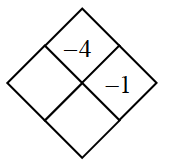•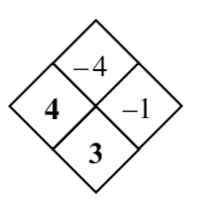1.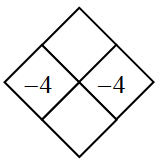•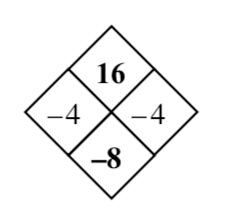1.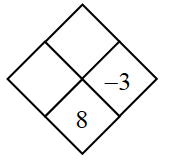• What plus $−3$ equals $8$?
What does $−3$ multiplied by that number equal?

1.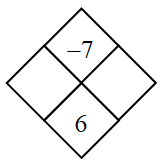• What are the factors of $−7$? Which two added together equal $6$?